# Accelerating TSNE with GPUs: From hours to seconds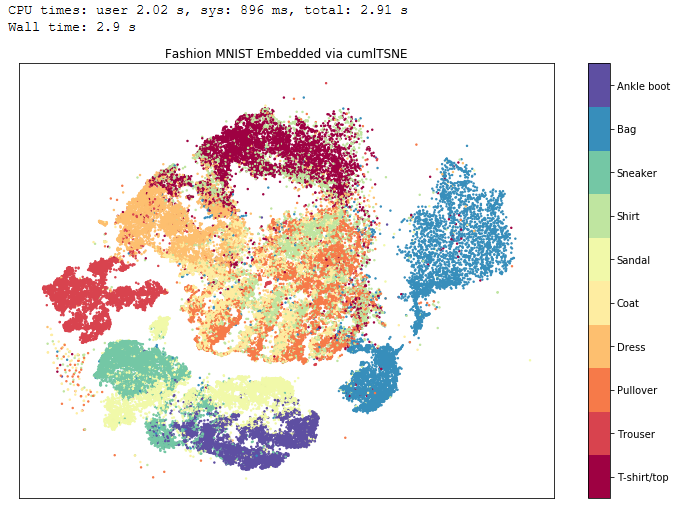Figure 1. cuML TSNE on MNIST Fashion takes 3 seconds. Scikit-Learn takes 1 hour.

TSNE (T-Distributed Stochastic Neighbor Embedding) is a popular unsupervised dimensionality reduction algorithm that finds uses as varied as neurology, image similarity, and visualizing neural networks. Unfortunately, its biggest drawback has been the long processing times in most available implementations. RAPIDS now provides fast GPU-accelerated TSNE, building on the GPU-based Barnes-Hut approach developed at CannyLab. TSNE in RAPIDS’ cuML machine learning library can run up to 2,000x faster than the corresponding CPU implementation (Scikit-Learn) and uses up to 30% less GPU memory than current GPU versions and can be 2x faster. (CannyLab’s BH TSNE).

This blog starts by presenting some example use cases, followed by benchmarks comparing cuML’s GPU TSNE implementation against scikit-learn. It then goes into a detailed explanation of how TSNE works and how it has been optimized in cuML to run on GPUs.

# Applications of TSNE

TSNE contrasts with traditional supervised methods like Linear Regression and Decision Trees, as it requires no labels. TSNE tries to discern structure in the data by moving points that are similar together and dis-similar points away from each other.

In Figure 2 above, TSNE is being applied to a fashion dataset that consists of 60,000 images of articles of clothing. This is useful for finding a natural grouping that will put “similar” garments close together. TSNE is able to reduce the complex space of fashion images to a smaller space, which is easier to work with. The vectors of pixels for each image are used as input and TSNE maps them to 2 dimensions, or 2 values for each image. In Figure 5, the 2-dimensional output from TSNE is plotted and color-coded according to the clothing category of the original input (e.g. boots are blue). TSNE is unaware of these categories, but finds a grouping that is able to put more similar items closer together.

Here is another example using the MNIST digits dataset. Given handwritten digits, the task is to classify each digit as 0, 1, 2 etc. After applying TSNE on all 60,000 images of digits, we find that without any labels, TSNE manages to separate the data. You can see in Figure 3 how there are clear clusters color-coded by digit type (0 to 9).

TSNE is also used to visualize Convolutional Neural Nets to help practitioners discern whether complex classifiers are actually “learning.” Below shows TSNE applied to AlexNet, where the output of the CNN of images before the actual classifier (4096 dimensions) is reduced to 2 dimensions, then visualized with the actual input image. Notice in Figure 4, similar images tend to be close, which implies how AlexNet “sees” them as being similar.

# TSNE vs. Principal Component Analysis (PCA)

TSNE is a nonlinear dimensionality reduction algorithm, whilst Principal Component Analysis is linear. This mean’s PCA’s components often have some meaning, while TSNE’s are no longer ordered by importance, or at all interpretable outside of the neighborhoods they create. On the CPU, it’s often recommended to reduce dimensions using PCA to 50 before feeding into TSNE for performance improvements. This is not the case for GPUs.

# RAPIDS cuML Speed-Up over Scikit-Learn

Many data scientists start with the popular TSNE implementation from scikit-learn. Scikit-learn’s TSNE (single threaded) provides a familiar, easy to use interface, but can run into scalability issues. For instance, a 60,000 example dataset could take 1 hour to converge in scikit-learn on CPU. The cuML TSNE implementation running on an NVIDIA V100 GPU can finish in 3 seconds on that same dataset.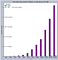Table 1. cuML’s TSNE time running on an NVIDIA DGX-1 with using 1 V100 GPU.

Notice the log scale in Table 1.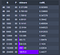Table 2. The timings in seconds between cuML and Scikit-Learn (DGX 1)

So cuML’s TSNE runs 1,000x faster, and it also attains similar trustworthiness scores.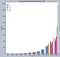Table 3. The full graph showcasing speedup of cuML over scikit-learn running on NVIDIA DGX 1.

On a dataset with 204,800 samples and 80 features, cuML takes 5.4 seconds while Scikit-learn takes almost 3 hours. This is a massive 2,000x speedup. We also tested TSNE on an NVIDIA DGX-1 machine using only one V100 GPU (DGX1: 32gb GV100 GPU, Intel Xeon E5–2698 v4 CPU@ 2.20GHz w/20 cores & 40 threads). The data transfer times were also included in this benchmark. Figure 5 shows a dataset containing 100 samples and 80 columns. Notice how cuML can be faster even on small datasets. Furthermore, RAPIDS TSNE is also around 200x faster than multicore TSNE.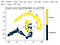Figure 5. cuML TSNE on the small Breast Cancer Data (1 second)

Using the PCA trick described above does give scikit-learn’s TSNE a slight boost in end-to-end performance, however, RAPIDS cuML TSNE is still demonstrating more than 1,000x speedup on tall datasets with 204,800 samples and 50 columns. This enables TSNE to be trained on datasets without first having to reduce the dimensions using PCA.

# How TSNE Works

cuML’s TSNE is based largely on CannyLab’s original Barnes Hut implementation. Currently, two algorithms are supported: Barnes Hut TSNE and Exact TSNE. Barnes Hut runs much faster than the Exact version, but is very slightly less accurate (at most 3% error). For large datasets (samples >= 2,000), the Barnes Hut algorithm is recommended for superior speed.

TSNE has 2 key objectives:

1. Close points should remain close.
2. Far points should remain distant.

Given some data points in a high dimensional setting (say 3D or 1,000 D), the goal is to embed the points in a lower space (eg. 2 dimensions), such that the local neighborhood structure of the input data is preserved as much as possible in its embedded form.

More concretely, points in the original high-dimensional space are first converted to probability densities that look like a bell curve, or normal distribution, like the red line in Figure 6 below. Points that are close increase each other’s probabilities, and so dense areas tend to have higher values. Likewise, outliers and dissimilar points have small values.

Now here’s where the “T-Distributed” part in TSNE’s name comes in. The points in the lower space are also modeled using a bell curve, though one which is stretched out like the blue line in Figure 6.

People have tried using a non-stretched version, but this causes a problem known as the “Crowding Problem”, where the embedded points clump together.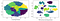Figure 7. Left showcases the crowding problem. TSNE solves this by using the T-Distribution.

Now, imagine springs connecting every point in the low dimensional space to every other point. Imagine the following scenario:

1. Points which are close originally will tug on each other. (Attraction)
2. Points which are dis-similar originally will push on each other. (Repulsion)

Essentially our spring’s function has been reversed. One would expect that far away points will cause the spring to pull them together, but in TSNE it’s the opposite.

Now let the springs in the low dimensional space be free. This is the optimization phase for TSNE. We terminate the evolving system when all the springs stop moving. We remove the springs, and the ending positions of each point becomes the final embedding.

# TSNE Optimizations

There are four optimizations used to improve the performance of TSNE on GPUs:

1. calculating higher dimensional probabilities with less GPU memory,
2. approximating higher dimensional probabilities,
3. reducing arithmetic operations, and

## Optimization 1 — Calculating Higher Dimensional Probabilities with less GPU Memory

Remember the goal to calculate the probabilities of each point by considering every other point’s influence? When the influence of point A to point B is not the same as point B on A, they are not symmetric. To make them equal, both contributions are summed up and divided between them. This is called symmetrizing the probabilities.

Originally, the symmetrization step was inefficient due to the use of unnecessary intermediate memory buffers. In the RAPIDS implementation, memory usage was reduced by 30% and is now highly parallelized. In total running times, symmetrization now takes 1%, or less of total elapsed times, as compared to 25% previously.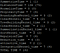Table 4. Timings of each kernel on the GPU. Symmetrization takes 1% of total time.

To implement this optimization, we first converted the distances between points into a COO (Coordinate Format) Sparse Matrix using fast cuML primitives. Sparse matrix formats are good at representing graphs of connected nodes and edges. This is especially true in the case of k-nearest neighbors graphs, which have a fixed number of connected edges, since only the closest neighbors of each point needs to be considered. Sparse formats only require the connected vertices to be stored, providing a significant speedup and lower storage overhead for algorithms like TSNE. The COO format is represented by 3 very simple arrays — the data values (COO_Vals), the column indices (COO_Cols), and the individual row indices (COO_Rows).

As an example, let’s say there’s a given point (0, 7) with value 10. It’s transpose (or reverse) is (7, 0), also with value 10. Here’s how to store this in the final COO sparse matrix:

To get its transpose or reverse, simply flip the col and row pointers like this:

Notice how the example above also includes an array named “RowPointer”. The COO layout does not include information about where each row starts or ends. Including this information allows us to parallelize lookups and quickly sum the transposed values in the symmetrization step. This RowPointer idea comes from the CSR (Compressed Sparse Row) Sparse Matrix layout. In the CSR layout, entries are indexed by which rows they are in. For example, all elements with row index 1 are placed together, in sorted order, at the start of the RowPointer index. The CSR layout is excellent for algorithms where data is accessed in a row-wise fashion.

Combining these two layouts allows us to use the COO format for efficient parallel computations over each element in the graph, while the CSR format is used to perform the transpose of the elements. Since the RowPointer contains the number of elements present in each row, the contributions of each pair of points can be summed in parallel using atomicAdd.

Figure 8 shows the whole process. Given the point (0, 7) with value 10, index the Row Pointer to get the row index for the point, and store it. Then, flip to (7, 0), access the Row Pointer, and store this as well in parallel with the first.

## Optimization 2 — Approximating Higher Dimensional Probabilities

It has been noted by van der Maaten, the author of TSNE that instead of computing full distances between all points, one can compute the top nearest neighbors and calculate the high dimensional probabilities from them. cuML followed CannyLabs’ approach of using Facebook’s FAISS library to compute the top-k neighbors on the GPU. This reduces the probability computation from having to store elements to storing only N*k elements (N is the number of data samples and k is the number of neighbors.)

## Optimization 3 — Reduce Arithmetic Operations

In many TSNE implementations, the attractive force computation (the spring tugging) is split to first be computed on point A and then on point B. TSNE can be made significantly faster if one computes the interaction, not separately, but at the same time. This reduces the number of multiplications and addresses from originally 9 to around 4, and makes this computation 50% faster.

## Optimization 4 — Broadcast along rows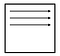Figure 9. Calculate common values and distribute it across each row!

Another fundamental optimization is noticing distances between point A in dimension 1 and dimension 2 are repeated across rows. This means instead of separately computing values for each dimension, compute it once, then broadcast and re-use the computation for the other dimension. This once again reduces arithmetic operations, and further speeds TSNE up. This is a general technique used by many CUDA algorithms, including many in cuML.

# Improving TSNE’s Numerical Stability

cuML has fixed some rare issues with numerical stability in CannyLab’s original implementation, including some infinite loops and out of bounds memory accesses. It is also known that TSNE is very sensitive to its hyperparameters. In cuML, an adaptive learning scheme is provided where parameters are adjusted based on the user’s input data.

Sometimes if the learning rate is too large, embedded points can become outliers. In cuML, a MAX_BOUND is specified which carefully pushes the outlier back and resets all momentum variables. This also helps improve TSNE’s precision and trustworthiness.

# How Do We Run TSNE in RAPIDS?

Let’s compare scikit-learn’s API to RAPIDS cuML’s API. This example uses the scikit-learn’s digits dataset.

scikit-learn API:

Now compare it with cuML:

Since cuML is a near drop-in replacement for scikit-learn, the “sklearn.manifold” package can be replaced with “cuml.manifold” and everything else will just work.

Here’s a Jupyter Notebook showing a demo of cuML TSNE on Fashion MNIST.

For more TSNE examples and a deeper dive into the mathematical optimizations on TSNE, check out a more extended Jupyter Notebook here.

# Conclusion

TSNE has been very successful at enabling the visualization of very large and complex datasets. It is able to discern structure in datasets without labels. Unfortunately, its biggest drawback has been its slow execution time.

With the new RAPIDS TSNE implementation, speedups up to 2,000x can be achieved while also using 30% less GPU memory. See what you think and provide feedback. Try out the free cuML TSNE for yourself on a Google Colab instance here.

# References for TSNE

David M. Chan, Roshan Rao, Forrest Huang, John F. Canny : t-SNE-CUDA: GPU-Accelerated t-SNE and its Applications to Modern Data https://arxiv.org/pdf/1807.11824.pdf [31 Jul 2018]

George C. Linderman, Manas Rachh, Jeremy G. Hoskins, Stefan Steinerberger, Yuval Kluger: Efficient Algorithms for t-distributed Stochastic Neighborhood Embedding https://arxiv.org/abs/1712.09005 [25 Dec 2017]

Laurens van der Maaten, Geoffrey Hinton : Visualizing High-Dimensional Data Using t-SNE https://lvdmaaten.github.io/publications/papers/JMLR_2008.pdf 

## RAPIDS AI

RAPIDS is a suite of software libraries for executing end-to-end data science & analytics pipelines entirely on GPUs.

Written by

## Daniel Han-Chen

I love to make machine learning algorithms faster! I’ve helped make NVIDIA RAPIDS TSNE run 2,000x faster than Sklearn, taking 5 seconds compared to 3 hours!## RAPIDS AI

RAPIDS is a suite of software libraries for executing end-to-end data science & analytics pipelines entirely on GPUs.